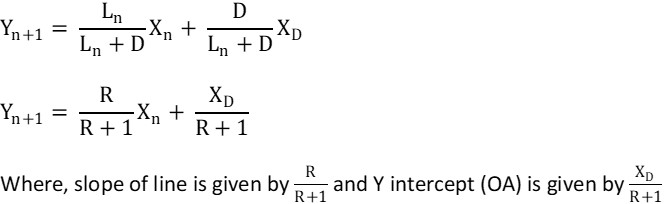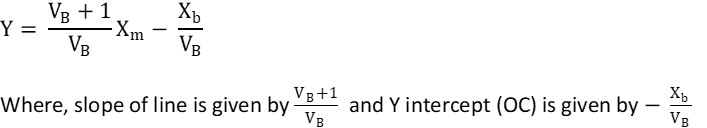# Material Balance around Operating Line

In any balance in the system, we have

Accumulation = input – output + generation – consumption……… (i)

However, while performing material balance in distillation column, we assume,

1. Operation is carried out in steady state (i.e. constant volume) ⇒ Accumulation = 0
2. We assume there is no reaction between components. ⇒ Generation = 0, Consumption = 0

Thus equation i now becomes

0 = input – output + 0 + 0

Input = output…….. (ii)

Because there are two sections in the column, there are two operating lines

1. Operating line: Rectifying section
2. Operating line: stripping section

Material balance around stripping section:

Rectifying operating line represents upper section of column. In the above figure, line AB represents operating line of rectifying section which follows the equation,Stripping operating line represents lower section of column. In the above figure, line AB represents operating line of stripping section which follows the equation,